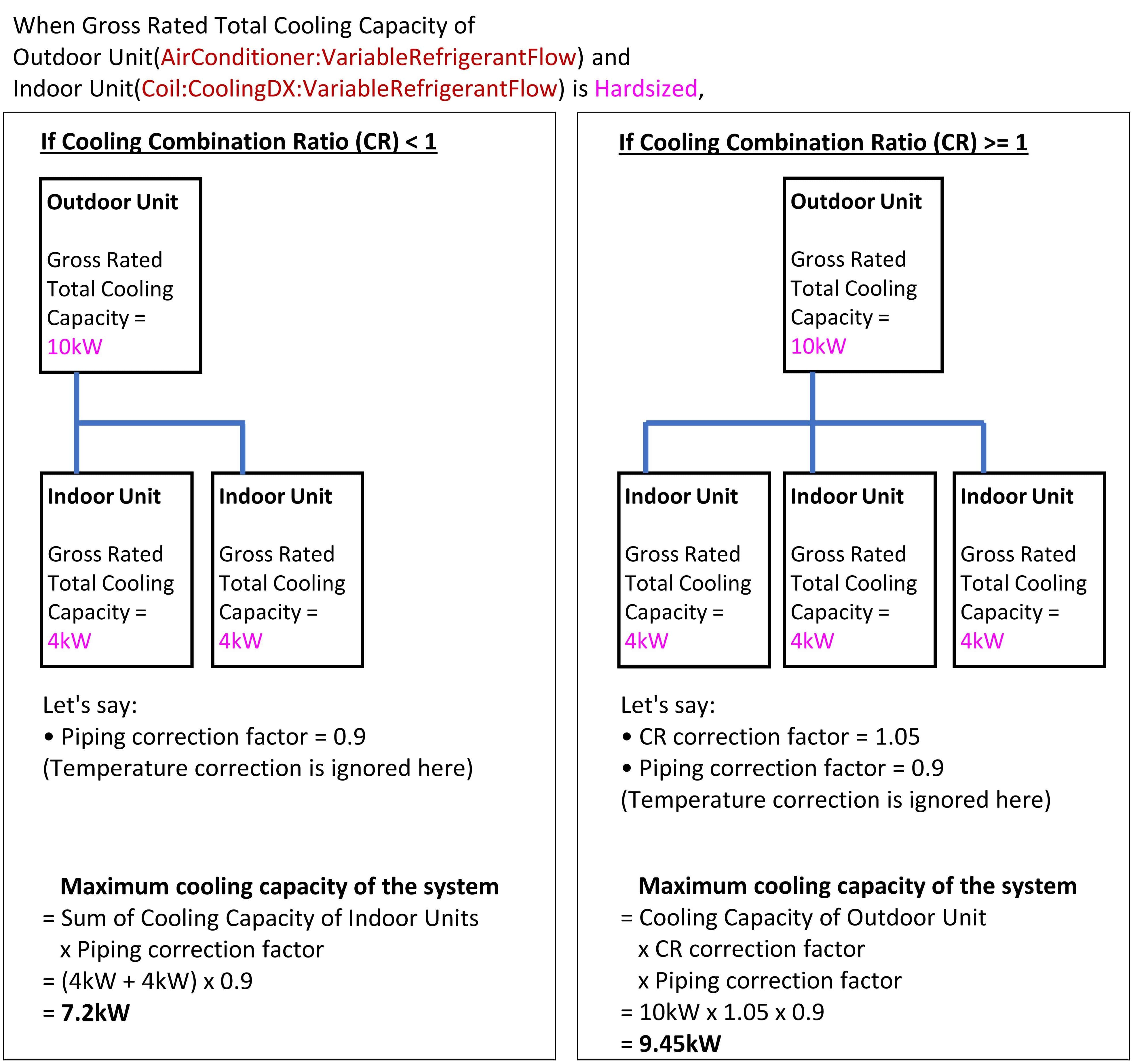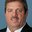Question-and-Answer Resource for the Building Energy Modeling Community
Get s tarted with the Help page

# Does Piping Correction Factor affect Hardsized cooling capacity of VRF?

This is a simple Yes or No question.

When we hardsizeGross Rated Total Cooling Capacity[W] in AirConditioner:VariableRefrigerantFlow, do Equivalent Piping Length used for Piping Correction Factor in Cooling Mode and Vertical Height used for Piping Correction Factor change the actual cooling capacity by correcting the hardsized Gross Rated Total Cooling Capacity? Or are they only relevant when Gross Rated Total Cooling Capacity is autosized?

For example, if Gross Rated Total Cooling Capacity is 10kW and Piping Correction Factor is 0.9, is the actual cooling capacity 10kW x 0.9 = 9kW? Or is it still 10kW?

Or to rephrase my question, when we hardsize Gross Rated Total Cooling Capacity[W] in AirConditioner:VariableRefrigerantFlow, should we input the cooling capacity taking into account the piping correction factor in advance? If the rated total cooling capacity on VRF product catalogue is 10kW and the piping correction factor that is manually calculated is 0.9, should we input 10kW x 0.9 = 9kW to Gross Rated Total Cooling Capacity?

P.S.

This postscript illustrates my interpretation of @rraustad 's answer. Could you please correct me if I'm wrong? I think the maximum cooling capacity of VRF system will be either Sum of the cooling capacity of indoor units or the Maximum cooling capacity of outdoor unit.

If sum of the cooling capacity of indoor units is smaller than the cooling capacity of outdoor unit, which means Combination Ratio (CR) is less than 1, then the maximum cooling capacity of the VRF system is sum of the cooling capacity of indoor units multiplied by Piping correction factor.

If sum of the cooling capacity of indoor units is larger than the cooling capacity of outdoor unit, which means Combination Ratio (CR) is more than 1, then the maximum cooling capacity of the VRF system is the cooling capacity of the outdoor unit multiplied by CR correction factor and Piping correction factor.edit retag close merge delete

Sort by » oldest newest most votedFrom a review of the VRF model it does not look like piping correction factor is used during sizing. This appears to be an oversight on sizing VRF systems. The piping correction factor adjusts the total terminal unit (TU) capacity (sum of all zone cooling coil capacity) as Total TU Cooling Capacity = Condenser Cooling Capacity * Piping Correction Factor. So in your example you should divide by piping correction factor as 10 kW / 0.9 = 11.11 kW. Then Total TU Cooling Capacity = 11.11 * 0.9 = 10 kW. The more piping losses there are, the larger the condenser needs to be to serve all the zone TUs.

more

Thank you for your answer. I understood that Piping correction factor is used to adjust the total cooling capacity of VRF after users hardsized the rated cooling capacity. However, I'm a bit skeptical that the piping correction factor always adjusts "the total TU capacity (sum of all zone cooling coil capacity)" because the total TU capacity is sometimes much larger than the capacity of the outdoor unit. In that case, the maximum cooling capacity of the VRF system is not the sum of capacity of indoor units. I added an illustration to my Question. Could you correct me if I'm wrong?

For CR > 1, if you sum up all the TU capacity you can't have more than 9.45 kW. The point was, if you want to size the condenser to meet the rated capacity of the TU's then condenser sizing must account for piping losses. Otherwise the TU capacity will be reduced.

Also note the combination ratio (CR) is a design concept and is only used during the simulation to adjust (using a constant determined at the beginning of the simulation during sizing) the system capacity based on the "design CR". For example, if you have 15 kW of TUs and 10 kW of condenser capacity then CR will be something >= 1 (e.g., 1.05). See manufacturers charts.